# TECH SPOT: SAMPLE CRE QUESTIONS (Part 19)1. The type of stress‐strength analysis when you have 3 or more variables in design for reliability is an example of:
A. Deterministic design
B. Probabilistic design
C. Data mining design
D. Design for maintainability

2. A bolted joint has shear strength which is normally distributed with mean, 40 klb/in2, and standard deviation, 4 k‐lb/in2. The joint is loaded with a shear stress that follows a normal distribution with mean 37 k‐lb/in2, and standard deviation of 3 k‐lb/in2. Find the approximate probability of failure of this bolted joint.
A. 0.73
B. 0.60
C. 0.43
D.0.27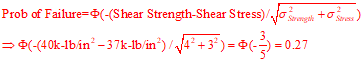3. In an FMECA, the item is a critical electrical component with mission time of 72 hours, and item failure rate λp of 10 per 106 hrs. The failure mode of the component is due to an overstress voltage condition. The failure model ratio, α, is 0.50, and the failure effect probability, β, is 1.00. An estimate of the failure rate of this failure mode is:

A. 36 per 106 hrs
B. 5 per 106 hrs
C. 360 per 106 hrs
D. 10 per 106 hrs

4. An assembly consists of three components in series.
Using three standard deviations and statistical tolerancing, what should be the tolerance for the overall length, 9.000 inches, of the assembly?
A. ± 0.007 inch
B. ± 0.012 inch
C. ± 0.021 inch
D. ± 0.027 inch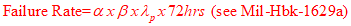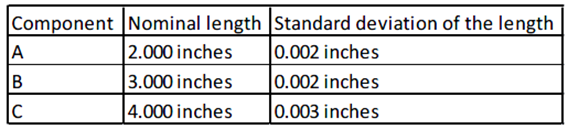5. Given a larger‐the‐better quality characteristic, a Taguchi experiment was run on 3 factors ‐ A, B, & C ‐ each held at two levels: Low & High. Both the mean response and a Taguchi signal‐to‐noise performance measures are summarized in the effects
plots below. Based on this information, which conclusion is the most correct?
A. Hold all 3 factors High
B. Hold all 3 factors Low.
C. Hold factors A and C high; hold factor B low.
D. Hold factor A high, and factors B & C low.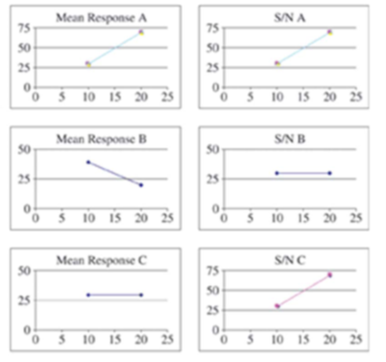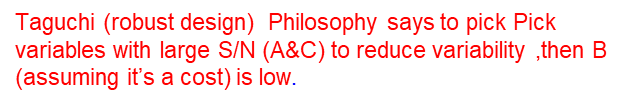6. If an equipment operator makes an error 10% of the time, and is operating equipment with reliability of 95%, which of the following best estimates the reliability of the human‐machine system? Assume the two errors are mutually independent.
A. 95%
B. 85%
C. 90%
D. 99%7. You have just completed 1000 tests at altitude with four failures of the Augmentor to light on the first try. Your customer comes in and asks for your progress in reliability demonstration and informs you the Chief Engineer of his company wants to have at least a Reliability=0.95 that the augmentor will light on first try. What can you tell him?
You demonstrated with 90% confidence which of thesee:
A. 0.971 reliability
B. 0.893 reliability
C. 0.93 reliability

D. 0.992 reliability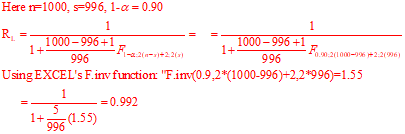8. Suppose that “bugs” are detected and corrected in developmental software at 1.4, 6.9, 24.3, 66.1, 117.2, and 229.3 hrs. %Calculate the expected number of failures by 1000 hours.
A. 6.2
B. 7.5
C. 9.0
D. 10.5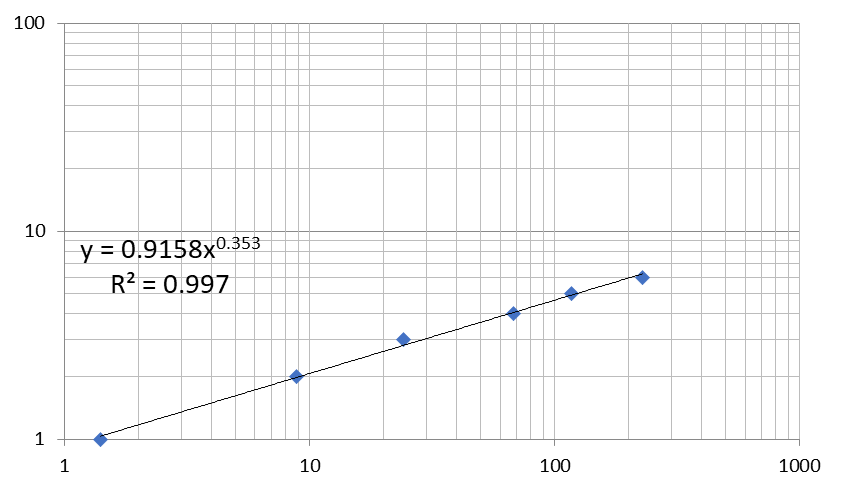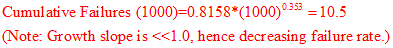9.Suppose that a device undergoing accelerated testing can be described by a Weibull distribution with a shape factor of β = 2.0. Under accelerated test conditions, with an acceleration factor (AF) = 5.0, 50% of the devices are found to fail during the first month. Under normal operating conditions, estimate how long the device will last before the failure probability reaches 10%. (the B10 life of the device).
A. 2 months
B. 3 months
C. 4 months
D. 6.5 months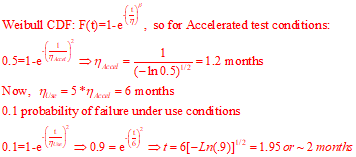10. Sets of electronic components are tested at 100°F and 120°F and the MTTFs are found to be 80 hr and 35 hr, respectively. Assuming that the Arrhenius equation is applicable, estimate the MTTF at 70°F. Assume a constant failure rate.
A. 100.2hrs
B. 220.5 hrs
C. 310.4hrs
D. 401.2 hrs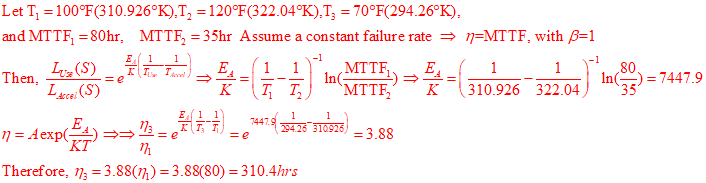## More To ExploreSample Questions

### TECH SPOT: SAMPLE CRE QUESTIONS (Part 21)

1. A system made up of 5 series components has a design reliability of .96. Subsequently, 4 of the components have reliabilities apportioned to them of 0.992, 0.991, 0.994, and 0.992. What should the reliability apportionment for the 5th subsystem be?A. 0.970 B. 0.969 C. 0.990 D. 0.992   2. Which process has as itsSample Questions

### TECH SPOT: SAMPLE CRE QUESTIONS (Part 20)

1. Which of the following is most valuable in Human engineering?: A.FMECA B. Digital computer C. Monte Carlo Analysis D. Full scale model 2. Hazard probability level “A” assigned to a hazard normally means: The Hazard ….. A. Is possible B. Is unlikely to occur C. Is very likely to occur D. Will occur sometimes

Scroll to Top